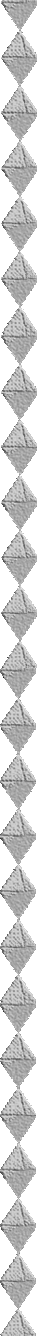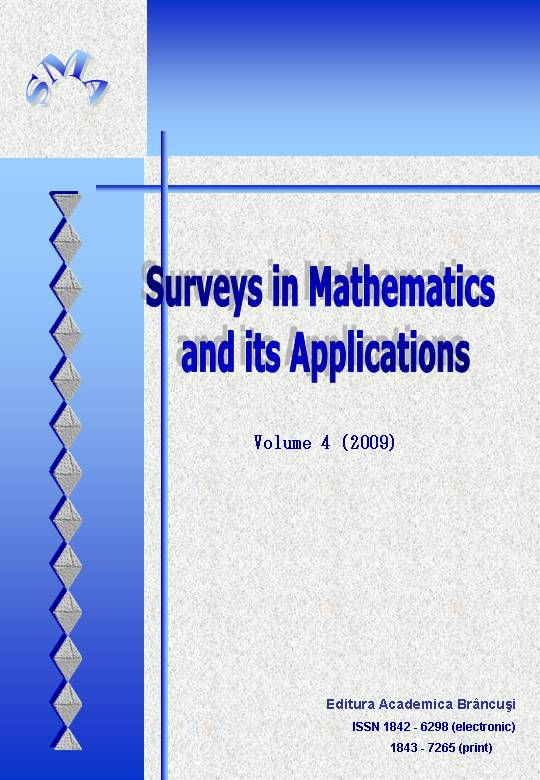## Volume 4 (2009)ISSN 1842-6298 (electronic)
ISSN 1843-7265 (print)

1. F. Wang, Y. Cui and F. Zhang, Existence and nonexistence results for second-order Neumann boundary value problem,
abstract:  pdf  html      full text:  pdf
1
2. S. Baghli, M. Benchohra and K. Ezzinbi, Controllability results for semilinear functional and neutral functional evolution equations with infinite delay,
abstract:  pdf  html      full text:  pdf
15
3. P. N. Dutta, B. S. Choudhury and K. P. Das, Some Fixed point results in Menger spaces using a control function,
abstract:  pdf  html      full text:  pdf
41
4. H. A. Jalab and R. W. Ibrahim, Almost-periodic solution for BAM neural networks,
abstract:  pdf  html      full text:  pdf
53
5. Mohd. Rafi and B. Lafuerza-Guillén, Probabilistic norms and statistical convergence of random variables,
abstract:  pdf  html      full text:  pdf
65
6. A. R. S. Juma and S. R. Kulkarni, Applications of generalized Ruscheweyh derivative to univalent functions with finitely many coefficients,
abstract:  pdf  html      full text:  pdf
77
7. L. Guran, Multivalued Perov-type theorems in generalized metric spaces,
abstract:  pdf  html      full text:  pdf
89
8. F. Batah and S. D. Gore, Ridge regression estimator: combining unbiased and ordinary ridge regression methods of estimation,
abstract:  pdf  html      full text:  pdf
99
9. R. W. Ibrahim, M. Darus and S. Momani, Subordination and superordination for certain analytic functions containing fractional integral,
abstract:  pdf  html      full text:  pdf
111
10. I. K. Argyros and S. Hilout, Enclosing roots of polynomial equations and their applications to iterative processes,
abstract:  pdf  html      full text:  pdf
119
11. A. K. Shukla and J. C. Prajapati, On a recurrence relation of generalized Mittag-Leffler function,
abstract:  pdf  html      full text:  pdf
133
12. M. H. Farag, Computing optimal control with a quasilinear parabolic partial differential equation,
abstract:  pdf  html      full text:  pdf
139
13. M. I. Alheety and B. M. Golam Kibria, On the Liu and almost unbiased Liu estimators in the presence of multicollinearity with heteroscedastic or correlated errors,
abstract:  pdf  html      full text:  pdf
155
14. L. Jäntschi and S. D. Bolboacă, Entropy due to Fragmentation of Dendrimers,
abstract:  pdf  html      full text:  pdf
169
15. X. Duan, Z. Peng and F. Duan, Positive definite solution of two kinds of nonlinear matrix equations,
abstract:  pdf  html      full text:  pdf
179
16. T.-L. Costache, On irreducible projective representations of finite groups,
abstract:  pdf  html      full text:  pdf
191
17. R. K. Bose and M. K. Roychowdhury, Fixed point theorems for generalized weakly contractive mappings,
abstract:  pdf  html      full text:  pdf
215
18. S. K. Upadhyay, R. N. Yadav and L. Debnath, The n-dimensional continuous wavelet transformation on Gelfand and Shilov type spaces,
abstract:  pdf  html      full text:  pdf
239
http://www.utgjiu.ro/math/smaWWW www.utgjiu.ro/math/sma